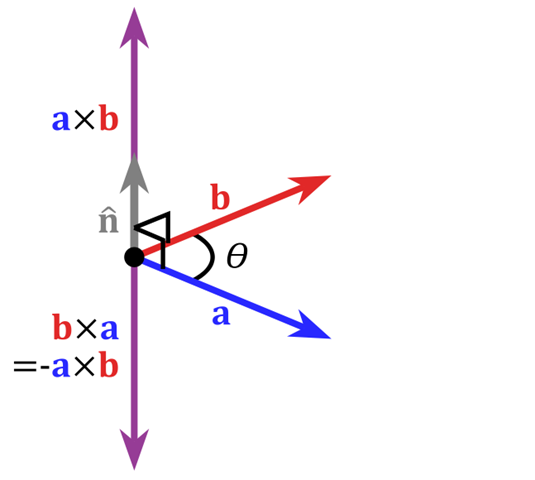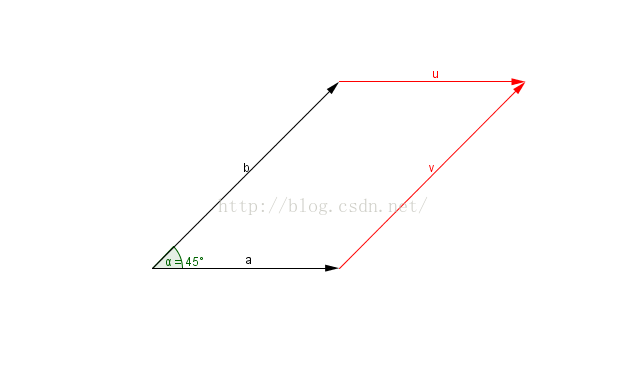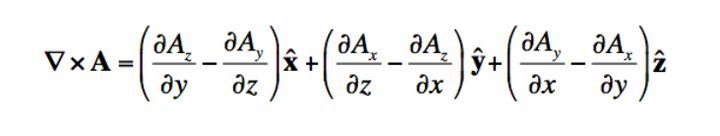• 哈密顿算子 点乘 叉乘 1、定义与性质 哈密顿算子：（数学符号：∇\nabla∇（又称nabla，奈布拉算子）），读来作Hamilton。 向量微分算子：∇=∂∂xi⃗+∂∂yj⃗+∂∂zk⃗\nabla=\frac{\partial }{\partial x}\vec{i}...
哈密顿算子 点乘 叉乘
1、定义与性质
哈密顿算子：（数学符号：$\nabla$（又称nabla，奈布拉算子）），读来作Hamilton。
向量微分算子：$\nabla=\frac{\partial }{\partial x}\vec{i}+\frac{\partial}{\partial y} \vec{j}+\frac{\partial }{\partial z}\vec{k}$
性质：

矢量性
微分算子
只对算子$\nabla$右边的量发生微分作用

麦克斯韦方程的微分形式：
$\frac{\partial D_{x}}{\partial x}+\frac{\partial D_{y}}{\partial y}+\frac{\partial D_{z}}{\partial z}=\rho$$\frac{\partial B_{x}}{\partial x}+\frac{\partial B_{y}}{\partial y}+\frac{\partial B_{z}}{\partial z}=0$
$\frac{\partial H_{z}}{\partial y} -\frac{\partial H_y}{\partial z} = \delta_{x}+\frac{\partial D_{x}}{\partial t}$$\frac{\partial H_{x}}{\partial z}-\frac{\partial H_{z}}{\partial x}=\delta_{y}+\frac{\partial D_{y}}{\partial t}$$\frac{\partial H_{y}}{\partial x}-\frac{\partial H_{x}}{\partial y}=\delta_{z}+\frac{\partial D_{z}}{\partial t}$
$\frac{\partial E_{z}}{\partial y}-\frac{\partial E_{y}}{\partial z}=-\frac{\partial B_{x}}{\partial t}$
$\frac{\partial E_{x}}{\partial z}-\frac{\partial E_{z}}{\partial x}=-\frac{\partial B_{y}}{\partial t}$
$\frac{\partial E_{y}}{\partial x}-\frac{\partial E_{x}}{\partial y}=-\frac{\partial B_{z}}{\partial t}$
引进哈密顿算子，上式简化为：
$\begin{cases} \nabla \cdot \vec{D}=\rho \\ \nabla \cdot \vec{B}=0 \\ \nabla \times \vec{H}=\vec{\delta}+\frac{\partial \vec{D}}{\partial t} \\ \nabla \times \vec{E}=-\frac{\partial \vec{B}}{\partial t} \end{cases}$
2、标量场的梯度
笛卡尔坐标系下的梯度：
$\nabla{P}=\frac{\partial P}{\partial x} \vec{i}+\frac{\partial P}{\partial y} \vec{j}+\frac{\partial P}{\partial z} \vec{k}=gradP$
（结果为矢量）
3、矢量场的散度
$\nabla \bullet \vec{V}=\frac{\partial V_{x}}{\partial x}+\frac{\partial V_{y}}{\partial y}+\frac{\partial V_{z}}{\partial z}=div \vec{V}$
（结果为标量）
4、矢量场的旋度
笛卡尔坐标系下旋度定义：
$\nabla \times \vec{V}=\left|\begin{array}{ccc} \vec{i} & \vec{j} & \vec{k} \\ \frac{\partial}{\partial x} & \frac{\partial}{\partial y} & \frac{\partial}{\partial z} \\ V_{x} & V_{y} & V_{z} \end{array}\right| = \left(\frac{\partial V_z}{\partial y} -\frac{\partial V_{y}}{\partial z}\right) \vec{i}+\left(\frac{\partial V_{x}}{\partial z}-\frac{\partial V_{z}}{\partial x}\right) \vec{j}+\left(\frac{\partial V_{y}}{\partial x}-\frac{\partial V_{x}}{\partial y}\right) \vec{k} = rot \vec{V}$
（结果为矢量）
5、哈密顿算子重要运算性质
$\nabla \cdot(\vec{A} \times \vec{B})=\vec{B} \cdot \nabla \times \vec{A}-\vec{A} \cdot \nabla \times \vec{B}$
6、向量内积与外积的性质与几何意义
向量内积的性质：

a^2 ≥ 0；当a^2 = 0时，必有a = 0. （正定性）
a·b = b·a. （对称性）
(λa + μb)·c = λa·c + μb·c，对任意实数λ, μ成立. （线性）
cos∠(a,b) =a·b/(|a||b|).
|a·b| ≤ |a||b|，等号只在a与b共线时成立.

内积（点乘）的几何意义包括：

表征或计算两个向量之间的夹角
b向量在a向量方向上的投影

向量外积的性质

a × b = -b × a. （反称性）
(λa + μb) × c = λ(a ×c) + μ(b ×c). （线性）

向量外积的几何意义
在三维几何中，向量a和向量b的外积结果是一个向量，有个更通俗易懂的叫法是法向量，该向量垂直于a和b向量构成的平面。
在3D图像学中，外积的概念非常有用，可以通过两个向量的外积，生成第三个垂直于a，b的法向量，从而构建X、Y、Z坐标系。如下图所示：在二维空间中，外积还有另外一个几何意义就是：|a×b|在数值上等于由向量a和向量b构成的平行四边形的面积。展开全文线性代数
• 旋度等于哈密顿算子叉乘向量，什么意思呢。首先我们从二维向量开始，二维的叉乘可以通过力矩的例子很形象：用力臂和力在垂直力臂方向上的分量的乘积表示力矩的大小，以右手定则规定力矩的方向——力矩就是描述关系的...
分享一些复习感悟。旋度等于哈密顿算子叉乘向量，什么意思呢。首先我们从二维向量开始，二维的叉乘可以通过力矩的例子很形象：用力臂和力在垂直力臂方向上的分量的乘积表示力矩的大小，以右手定则规定力矩的方向——力矩就是描述关系的算式。但是到了三维怎么就多了这么多的量？定义一个向量A，在xyz方向上的大小分别是Ax，Ay，Az，旋度如下：其实三维的叉乘就是计算三个方向上的“力矩”写到一起（数学上表示为加法）。看似花哨的式子其实很有道理，X方向上的“力矩”自然和x方向的“力”Ax无关，所以是Az和Ay。那么什么是偏导呢？偏导其实就是导数，但是由于实际上收到三个变量的影响，所以写成偏导为什么Az的结果要和Ay做差？显然两个方向是等价概念，但是因为设定上Z方向的力和Y方向的力对X方向的旋度贡献相反，所以我们使用右手定则的时候就取Y方向为负。其他方向同理
展开全文• 重要公式 ▽⋅(A⃗×B⃗)=B⃗⋅▽×A⃗−A⃗⋅▽×B⃗ \triangledown \cdot(\vec A \times \vec B)= \vec B \cdot \triangledown \times\vec A-\vec A\cdot\triangledown\times\vec B ▽⋅(A×B)=B⋅▽×A−A⋅▽×B...

重要公式
$\triangledown \cdot(\vec A \times \vec B)= \vec B \cdot \triangledown \times\vec A-\vec A\cdot\triangledown\times\vec B$
证明
$\triangledown=\frac{\partial}{\partial x}\vec e_x+\frac{\partial }{\partial y}\vec e_y+\frac{\partial }{\partial z}\vec e_z$
$\vec A \times \vec B=\left | \begin{matrix} \vec e_x &\vec e_y &\vec e_z\\ A_x & A_y & A_z\\ B_x & B_y & B_z \end{matrix} \right |\\ =(A_yB_z-A_zB_y)\vec e_x+(A_zB_x-A_xB_z)\vec e_y+(A_xB_y-A_yB_x)\vec e_z$
$\triangledown \cdot(\vec A \times \vec B)=(\frac{\partial}{\partial x}\vec e_x+\frac{\partial }{\partial y}\vec e_y+\frac{\partial }{\partial z}\vec e_z) \cdot\bigg((A_yB_z-A_zB_y)\vec e_x+(A_zB_x-A_xB_z)\vec e_y+(A_xB_y-A_yB_x)\vec e_z\bigg)\\ =\frac{\partial{(A_yB_z-A_zB_y)}}{\partial x}+\frac{\partial{(A_zB_x-A_xB_z)}}{\partial y}+\frac{\partial{(A_xB_y-A_yB_x)}}{\partial z}\\ =\left | \begin{matrix} \frac{\partial}{\partial x}&\frac{\partial }{\partial y}&\frac{\partial }{\partial z}\\ A_x & A_y & A_z\\ B_x & B_y & B_z \end{matrix} \right |\\$

$\vec B \cdot \triangledown \times\vec A-\vec A\cdot\triangledown\times\vec B=\vec B \cdot \left | \begin{matrix} \vec e_x &\vec e_y &\vec e_z\\ \frac{\partial}{\partial x}&\frac{\partial }{\partial y}&\frac{\partial }{\partial z}\\ A_x & A_y & A_z \end{matrix} \right | - \vec A \cdot \left | \begin{matrix} \vec e_x &\vec e_y &\vec e_z\\ \frac{\partial}{\partial x}&\frac{\partial }{\partial y}&\frac{\partial }{\partial z}\\ B_x & B_y & B_z \end{matrix}\right | \\ = (B_x\vec e_x+B_y\vec e_y+B_z\vec e_z)\cdot\bigg( (\frac{\partial A_z}{\partial y}-\frac{\partial A_y}{\partial z})\vec e_x+(\frac{\partial A_x}{\partial z}-\frac{\partial A_z}{\partial x})\vec e_y+(\frac{\partial A_y}{\partial x}-\frac{\partial A_x}{\partial y})\vec e_z \bigg)\\ -(A_x\vec e_x+A_y\vec e_y+A_z\vec e_z)\cdot\bigg( (\frac{\partial B_z}{\partial y}-\frac{\partial B_y}{\partial z})\vec e_x+(\frac{\partial B_x}{\partial z}-\frac{\partial B_z}{\partial x})\vec e_y+(\frac{\partial B_y}{\partial x}-\frac{\partial B_x}{\partial y})\vec e_z \bigg)\\ =B_x( \frac{\partial A_z}{\partial y}-\frac{\partial A_y}{\partial z})+ B_y(\frac{\partial A_x}{\partial z}-\frac{\partial A_z}{\partial x})+ B_z(\frac{\partial A_y}{\partial x}-\frac{\partial A_x}{\partial y})\\ -\bigg(A_x( \frac{\partial B_z}{\partial y}-\frac{\partial B_y}{\partial z})+ A_y(\frac{\partial B_x}{\partial z}-\frac{\partial B_z}{\partial x})+ A_z(\frac{\partial B_y}{\partial x}-\frac{\partial B_x}{\partial y})\bigg)\\ \because B_z\frac{\partial A_y}{\partial x}-B_y\frac{\partial A_z}{\partial x}+ A_y\frac{\partial B_z}{\partial x}- A_z\frac{\partial B_y}{\partial x}= \bigg(B_z\frac{\partial A_y}{\partial x}+ A_y\frac{\partial B_z}{\partial x}\bigg)-\bigg(B_y\frac{\partial A_z}{\partial x}+A_z\frac{\partial B_y}{\partial x} \bigg)\\ =\frac{\partial( A_yB_z)}{\partial x}-\frac{\partial( A_zB_y)}{\partial x}=\frac{\partial( A_yB_z-A_zB_y)}{\partial x}$

同理其他三项可得，证毕


展开全文• 重要公式 ▽⋅(A⃗×B⃗)=B⃗⋅▽×A⃗−A⃗⋅▽×B⃗ \triangledown \cdot(\vec A \times \vec B)= \vec B \cdot \triangledown \times\vec A-\vec A\cdot\triangledown\times\vec B ▽⋅(A×B)=B⋅▽×A−A⋅▽×B...

重要公式
$\triangledown \cdot(\vec A \times \vec B)= \vec B \cdot \triangledown \times\vec A-\vec A\cdot\triangledown\times\vec B$
证明
$\triangledown=\frac{\partial}{\partial x}\vec e_x+\frac{\partial }{\partial y}\vec e_y+\frac{\partial }{\partial z}\vec e_z$
$\vec A \times \vec B=\left | \begin{matrix} \vec e_x &\vec e_y &\vec e_z\\ A_x & A_y & A_z\\ B_x & B_y & B_z \end{matrix} \right |\\ =(A_yB_z-A_zB_y)\vec e_x+(A_zB_x-A_xB_z)\vec e_y+(A_xB_y-A_yB_x)\vec e_z$
$\triangledown \cdot(\vec A \times \vec B)=(\frac{\partial}{\partial x}\vec e_x+\frac{\partial }{\partial y}\vec e_y+\frac{\partial }{\partial z}\vec e_z) \cdot\bigg((A_yB_z-A_zB_y)\vec e_x+(A_zB_x-A_xB_z)\vec e_y+(A_xB_y-A_yB_x)\vec e_z\bigg)\\ =\frac{\partial{(A_yB_z-A_zB_y)}}{\partial x}+\frac{\partial{(A_zB_x-A_xB_z)}}{\partial y}+\frac{\partial{(A_xB_y-A_yB_x)}}{\partial z}\\ =\left | \begin{matrix} \frac{\partial}{\partial x}&\frac{\partial }{\partial y}&\frac{\partial }{\partial z}\\ A_x & A_y & A_z\\ B_x & B_y & B_z \end{matrix} \right |\\$

$\vec B \cdot \triangledown \times\vec A-\vec A\cdot\triangledown\times\vec B=\vec B \cdot \left | \begin{matrix} \vec e_x &\vec e_y &\vec e_z\\ \frac{\partial}{\partial x}&\frac{\partial }{\partial y}&\frac{\partial }{\partial z}\\ A_x & A_y & A_z \end{matrix} \right | - \vec A \cdot \left | \begin{matrix} \vec e_x &\vec e_y &\vec e_z\\ \frac{\partial}{\partial x}&\frac{\partial }{\partial y}&\frac{\partial }{\partial z}\\ B_x & B_y & B_z \end{matrix}\right | \\ = (B_x\vec e_x+B_y\vec e_y+B_z\vec e_z)\cdot\bigg( (\frac{\partial A_z}{\partial y}-\frac{\partial A_y}{\partial z})\vec e_x+(\frac{\partial A_x}{\partial z}-\frac{\partial A_z}{\partial x})\vec e_y+(\frac{\partial A_y}{\partial x}-\frac{\partial A_x}{\partial y})\vec e_z \bigg)\\ -(A_x\vec e_x+A_y\vec e_y+A_z\vec e_z)\cdot\bigg( (\frac{\partial B_z}{\partial y}-\frac{\partial B_y}{\partial z})\vec e_x+(\frac{\partial B_x}{\partial z}-\frac{\partial B_z}{\partial x})\vec e_y+(\frac{\partial B_y}{\partial x}-\frac{\partial B_x}{\partial y})\vec e_z \bigg)\\ =B_x( \frac{\partial A_z}{\partial y}-\frac{\partial A_y}{\partial z})+ B_y(\frac{\partial A_x}{\partial z}-\frac{\partial A_z}{\partial x})+ B_z(\frac{\partial A_y}{\partial x}-\frac{\partial A_x}{\partial y})\\ -\bigg(A_x( \frac{\partial B_z}{\partial y}-\frac{\partial B_y}{\partial z})+ A_y(\frac{\partial B_x}{\partial z}-\frac{\partial B_z}{\partial x})+ A_z(\frac{\partial B_y}{\partial x}-\frac{\partial B_x}{\partial y})\bigg)\\ \because B_z\frac{\partial A_y}{\partial x}-B_y\frac{\partial A_z}{\partial x}+ A_y\frac{\partial B_z}{\partial x}- A_z\frac{\partial B_y}{\partial x}= \bigg(B_z\frac{\partial A_y}{\partial x}+ A_y\frac{\partial B_z}{\partial x}\bigg)-\bigg(B_y\frac{\partial A_z}{\partial x}+A_z\frac{\partial B_y}{\partial x} \bigg)\\ =\frac{\partial( A_yB_z)}{\partial x}-\frac{\partial( A_zB_y)}{\partial x}=\frac{\partial( A_yB_z-A_zB_y)}{\partial x}$

同理其他三项可得，证毕


展开全文• 重要公式 ▽⋅(A⃗×B⃗)=B⃗⋅▽×A⃗−A⃗⋅▽×B⃗ \triangledown \cdot(\vec A \times \vec B)= \vec B \cdot \triangledown \times\vec A-\vec A\cdot\triangledown\times\vec B ▽⋅(A×B)=B⋅▽×A−A⋅▽...
• ∇=[∂∂x∂∂y∂∂z]，∇×=[0−∂∂z∂∂y∂∂z0−∂∂x−∂∂y∂∂x0]\nabla= \begin{bmatrix} \frac{\partial}{\partial x} \\\\ \frac{\partial}{\partial y} \\\\ \frac{\partial}{\partial z} ...
• 针对环量子引力，提出了一种新的对称哈密顿约束算子，该算子在微分不变态的希尔伯特空间直至价高于三的非平面顶点上得到了很好的定义。 它继承了原始正则化方法的优点，可以为自旋网络创建新的顶点。 该哈密顿量的...
• 一、微分算子 （一）哈密顿算子 在直角坐标系中，哈密顿算子定义为：基本概念
• 辛空间两类算子阵的谱分析，张东，杨雪源 ，本文讨论了辛空间中哈密顿算子矩阵及反哈密顿算子阵特征值和特征向量性质，证明了哈密顿阵的数值域关于Y轴对称，对于几类简单的�
• 对于均质解Rr2-1r2-1，构造了相应一维量子可积模型的哈密顿算子，该算子描述了不同类型的自旋态之间相当复杂的相互作用。 讨论了在复合状态的乘积上定义的扶正器运算符。 还提出了RrR（u）算子的不均匀序列，即...
• 你先记住哈密顿算子▽ 他表示一个矢量算子（注意）： 　▽≡i*d/dx+j*d/dy+k*d/dz 　运算规则: 　一、▽A=(i*d/dx+j*d/dy+k*d/dz)A=i*dA/dx+j*dA/dy+k*dA/dz 　这样标量场A通过▽的这个运算就形成了一个矢量场...
• 声明：由于笔者只是一名大一并且非数学系的本科生，因此以下内容基于自己的理解与参考了几...哈密顿算子本身并无意义，就是一个算子，同时又被看作是一个矢量，在运算时，具有矢量和微分的双重身份。 在二维坐标系下，
• Gradient Descent Δ\DeltaΔ 哈密顿算子 初始位置深度学习 python
• 重子特征值问题是使用Fock空间哈密顿算子提出的，该算子是通过将RGPEP方程求解至偶数次幂而获得的。 试探性地删除包含三个夸克和两个或多个胶子的本征态分量，代价是在一个胶子中插入一个胶子质量项。 对于三个夸克...
• 由神经网络的激活函数 ...只要假设f（x）与f（-x）分别是电场与磁场，并且假设哈密顿算子是一维并且 由这个方程组可以得到一维的哈密顿算子表达式 在进一步的假设x是时间t，把所有的这些假设综合就相当于...神经网络
• 最近复习电磁场与电磁波时，又学习了一遍场论基础，但是现有教程的概念比之前本科学习的谢处方老师的《电磁场与电磁波》的概念要难理解，另外哈密顿算子、拉普拉斯算子在图像方面也有重要应用，遂在此进行简单总结，...数学
• ## 基本矢量计算

千次阅读 2018-11-17 21:58:11
微分算子或哈密顿算子： 梯度： 散度： 旋度： 拉普拉斯算子： 二重点积： 高斯公式： 例子：将纳维斯托克斯方程矢量形式展开并获得直角坐标系下三维伸展方向的表达式 纳维斯托克斯...矢量 高斯公式
• 构造了二维至超三维渐近平面N = 1 ... 它是基于哈密顿算子中的超级庞加莱代数（分别是拉格朗日公式）的受约束或有序的手性Wess-Zumino-Witten动作来描述的，其减少的相空间描述对应于平坦Liouville理论的超对称扩展。
• 1.算子的定义首先定义一个向量算子 ： 该算子也叫哈密顿算子，其中 ， 和 分别是 ， 和 方向的单位向量。为了计算方便，我们引入线性代数的风格来改写公式（ 1），即： 其中， 表示转置。2.梯度首先说明，梯度是一个...
• 其中许多内容是作者多年来的研究成果，特别是提出完全泛函的极值函数定理，统一了变分法中的各种欧拉方程，创立含向量、向量的模、任意阶张量和哈密顿算子的泛函的变分理论，给出相应的欧拉方程组及自然边界条件，...
• 这几天查资料看到的，顺手写下来当做笔记拉梅系数...设 是可以推导的: , 运用公式： ，算出 得到： 此时也得到了该坐标系下的哈密顿算子 之后是散度，此时的 是矢量函数，可以写成 暴力计算后可以得到，但是不够优...r矢量球坐标系旋度
• 通常，将表示形式定义为对角化某些算子，例如，绝热表示形式将对角线化的哈密顿算子。 然而，当操作员具有退化特征值（接近）时，这可能会成为问题，因为相应的特征向量可以任意混合，从而将奇异性引入到基本向量的...C++
• 为了研究相关的统计力学，需要找到微状态的能量，该能量是聚合物框架中哈密顿算子的特征值。 但是，这根本不是一件容易的事，因为哈密顿量在聚合物图像中呈非线性形式。 在本文中，我们介绍了一种半经典方法，该...
• 场2.1 定义2.2 分类2.3 矢量场的通量2.3.1 定义2.3.2 公式2.4 矢量场的散度2.4.1 定义2.4.2 表达式2.4.3 哈密顿算子2.4.4 散度的性质2.4.5 散度定理（高斯定理）2.4.6 散度的应用3. 总结 1. 矢量 1.1 矢量表示 标量...
• 比如量子门，量子态，可观测量，本征态，希伯尔空间向量，薛定谔方程，哈密顿算子，量子比特等。 正文 -1. 简明量子力学 (第1讲) ：什么是量子？ 一些基本的知识，对量子力学的概述，了解 -2. 简明量子力学（第2...量子力学 算法 线性代数
• 量子哈密顿算子和对应的CQG状态和波函数的CQG波方程以4标量形式实现。 事实证明，新的量子波方程等效于一组量子流体动力学方程，该方程可保证与经典GR Hamilton-Jacobi方程在半经典范围内的一致性。 开发了一种扰动...
• 文章目录前言正文需要解决的问题和思路近似公式和向量内积多变量下的梯度下降法（重点）多变量下的梯度下降法（重点）哈密顿算子▽η的含义总结 前言 本篇主要讲解一个机器学习中很重要但又很基础的概念：梯度下降，...深度学习 神经网络
• 旋度公式记住公式好办你先记住哈密顿算子▽ 他表示一个矢量算子(注意)：▽≡i*d/dx+j*d/dy+k*d/dz运算规则:一、▽A=(i*d/dx+j*d/dy+k*d/dz)A=i*dA/dx+j*dA/dy+k*dA/dz这样标量场A通过▽的这个运算就形成了一个矢量场...
• 场的概念 | 方向导数与梯度 | 通量与散度 | 环量与旋度 | 典型矢量场 | 哈密顿算子场的概念1.场：如果在全部空间或部分空间里的每一点，都对应着某个物理量的一个确定的值，即在这个空间里确定了该物理量的一个场。...r矢量球坐标系旋度
• 我们证明任何非相对论的经典系统都必须服从与该系统的Schrödinger方程完全相同的统计波动方程，包括通常的“规范量化”和哈密顿算子，前提是未知常数设置为。 我们展示了为什么两个方程必须具有完全相同的解集，...